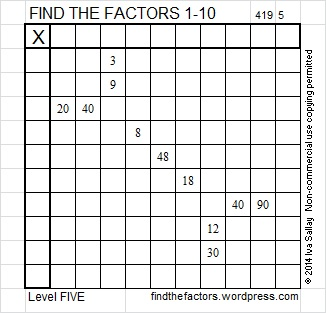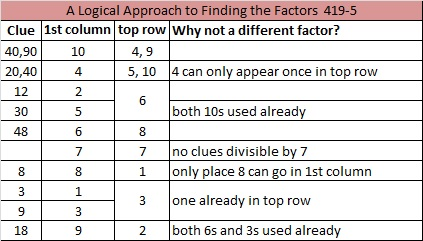# 120 and Level 5

120  is a composite number. 120 = 1 x 120, 2 x 60, 3 x 40, 4 x 30, 5 x 24, 6 x 20, 8 x 15, or 10 x 12. Factors of 120: 1, 2, 3, 4, 5, 6, 8, 10, 12, 15, 20, 24, 30, 40, 60, 120. Prime factorization: 120 = 2 x 2 x 2 x 3 x 5, which can also be written 120 = 2³ x 3 x 5.Thinking process using divisibility tricks to find the factor pairs of 120:

√120 is irrational and approximately equal to 10.95. Every factor pair of 120 will have one factor less than 10.95 and one factor greater than 10.95, and we will find both factors in each pair at the same time. The following numbers are less than 10.95. Are they factors of 120?

1. Yes, all whole numbers are divisible by 1, so 1 x 120 = 120.
2. Yes, 120 is an even number. 120 ÷ 2 = 60, so 2 x 60 = 120. (Since 60 is even, 4 will also be a factor of 120.)
3. Yes, 1 + 2 + 0 = 3 which is divisible by 3 (but not by 9), so 120 is divisible by 3. 120 ÷ 3 = 40, so 3 x 40 = 120. Note 120 will not be divisible by 9.
4. Yes, the number formed from its last two digits, 20, is divisible by 4, so 120 is divisible by 4, and 4 x 30 = 120. (Note since 30 is even, 8 will also be a factor of 120.)
5. Yes, the last digit is 0 or 5, so 120 is divisible by 5, and 5 x 24 = 120.
6. Yes, 120 is divisible by both 2 and 3, so it is divisible by 6, and 6 x 20 = 120.
7. No. The divisibility trick for 7 requires us to split 120 into 12 and 0. We double 0 and subtract the double from 12. 12 – (2 x 0) = 12 – 0 = 12. Since 12 is not divisible by 7, 120 also is not divisible by 7.
8. Yes, see 4 above. 120 = 8 x 15. (This will mean that ANY number whose last 3 digits are 120 will also be divisible by 8.)
9. No, see 3 above. 120 is not divisible by 9.
10. Yes, 120 ends with a zero, so 10 is a factor of 120, and 10 x 12 = 120.

From this thinking process we conclude that the factor pairs of 120 are 1 x 120, 2 x 60, 3 x 40, 4 x 30, 5 x 24, 6 x 20, 8 x 15, and 10 x 12.

When 120 is a clue in the FIND THE FACTORS 1 – 12 puzzles, use 10 and 12 as the factors.

5! = 1 x 2 x 3 x 4 x 5 = 120.Excel file of this week’s puzzles and last week’s factors: 10 Factors 2014-05-12Advertisements

## 6 thoughts on “120 and Level 5”

1.Steve Morris

You are making numbers very interesting!

•ivasallay

Thank you. If numbers are interesting or boring is always a personal decision. I like the decision you’ve made!

•Joseph Nebus

Oh, I like that attitude. There is almost always something interesting to be found in numbers, anyway.

2.ivasallay

So true. I’ve decided to add a link to each post with interesting facts about the factored number.

•Steve Morris

yay!

•Joseph Nebus

Yeah, the little facts are fun extras.

This site uses Akismet to reduce spam. Learn how your comment data is processed.